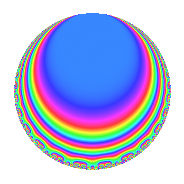# Properties

 Label 4032.2.b.jLevel 4032 Weight 2 Character orbit 4032.b Analytic conductor 32.196 Analytic rank 0 Dimension 4 CM No Inner twists 2

# Related objects

## Newspace parameters

 Level: $$N$$ = $$4032 = 2^{6} \cdot 3^{2} \cdot 7$$ Weight: $$k$$ = $$2$$ Character orbit: $$[\chi]$$ = 4032.b (of order $$2$$ and degree $$1$$)

## Newform invariants

 Self dual: No Analytic conductor: $$32.195682095$$ Analytic rank: $$0$$ Dimension: $$4$$ Coefficient field: 4.0.2312.1 Coefficient ring: $$\Z[a_1, \ldots, a_{7}]$$ Coefficient ring index: $$2^{2}$$ Sato-Tate group: $\mathrm{SU}(2)[C_{2}]$

## $q$-expansion

Coefficients of the $$q$$-expansion are expressed in terms of a basis $$1,\beta_1,\beta_2,\beta_3$$ for the coefficient ring described below. We also show the integral $$q$$-expansion of the trace form.

 $$f(q)$$ $$=$$ $$q + ( -1 + \beta_{1} + \beta_{2} + \beta_{3} ) q^{5} -\beta_{3} q^{7} +O(q^{10})$$ $$q + ( -1 + \beta_{1} + \beta_{2} + \beta_{3} ) q^{5} -\beta_{3} q^{7} + \beta_{2} q^{11} + 2 \beta_{2} q^{13} + ( -1 + \beta_{1} - \beta_{2} + \beta_{3} ) q^{17} + ( 3 + \beta_{1} - \beta_{3} ) q^{19} + \beta_{2} q^{23} + ( -2 - \beta_{1} + \beta_{3} ) q^{25} -2 q^{29} + ( 2 + 2 \beta_{1} - \beta_{2} ) q^{35} + ( 3 - \beta_{1} + \beta_{3} ) q^{37} + ( -1 + \beta_{1} + 3 \beta_{2} + \beta_{3} ) q^{41} + ( -3 + 3 \beta_{1} + 4 \beta_{2} + 3 \beta_{3} ) q^{43} + ( -2 + 2 \beta_{1} - 2 \beta_{3} ) q^{47} + ( 4 - 3 \beta_{1} - 2 \beta_{2} - \beta_{3} ) q^{49} + ( 4 + 2 \beta_{1} - 2 \beta_{3} ) q^{53} + ( -1 + \beta_{1} - \beta_{3} ) q^{55} -4 q^{59} + ( 2 - 2 \beta_{1} - 4 \beta_{2} - 2 \beta_{3} ) q^{61} + ( -2 + 2 \beta_{1} - 2 \beta_{3} ) q^{65} + ( 3 - 3 \beta_{1} - 2 \beta_{2} - 3 \beta_{3} ) q^{67} + ( 2 - 2 \beta_{1} - 5 \beta_{2} - 2 \beta_{3} ) q^{71} + ( -2 + 2 \beta_{1} + 2 \beta_{2} + 2 \beta_{3} ) q^{73} + ( -1 - \beta_{1} - 3 \beta_{2} - \beta_{3} ) q^{77} + ( -1 + \beta_{1} + 2 \beta_{2} + \beta_{3} ) q^{79} + ( 2 + 2 \beta_{1} - 2 \beta_{3} ) q^{83} + ( -5 - 3 \beta_{1} + 3 \beta_{3} ) q^{85} + ( 1 - \beta_{1} - 3 \beta_{2} - \beta_{3} ) q^{89} + ( -2 - 2 \beta_{1} - 6 \beta_{2} - 2 \beta_{3} ) q^{91} + ( -6 + 6 \beta_{1} + 2 \beta_{2} + 6 \beta_{3} ) q^{95} + ( 2 - 2 \beta_{1} + 2 \beta_{2} - 2 \beta_{3} ) q^{97} +O(q^{100})$$ $$\operatorname{Tr}(f)(q)$$ $$=$$ $$4q - 2q^{7} + O(q^{10})$$ $$4q - 2q^{7} + 12q^{19} - 8q^{25} - 8q^{29} + 12q^{35} + 12q^{37} - 8q^{47} + 8q^{49} + 16q^{53} - 4q^{55} - 16q^{59} - 8q^{65} - 8q^{77} + 8q^{83} - 20q^{85} - 16q^{91} + O(q^{100})$$

Basis of coefficient ring in terms of a root $$\nu$$ of $$x^{4} - x^{3} - 2 x + 4$$:

 $$\beta_{0}$$ $$=$$ $$1$$ $$\beta_{1}$$ $$=$$ $$\nu^{2} + \nu$$ $$\beta_{2}$$ $$=$$ $$-\nu^{2} + \nu$$ $$\beta_{3}$$ $$=$$ $$\nu^{3} - \nu - 1$$
 $$1$$ $$=$$ $$\beta_0$$ $$\nu$$ $$=$$ $$($$$$\beta_{2} + \beta_{1}$$$$)/2$$ $$\nu^{2}$$ $$=$$ $$($$$$-\beta_{2} + \beta_{1}$$$$)/2$$ $$\nu^{3}$$ $$=$$ $$($$$$2 \beta_{3} + \beta_{2} + \beta_{1} + 2$$$$)/2$$

## Character Values

We give the values of $$\chi$$ on generators for $$\left(\mathbb{Z}/4032\mathbb{Z}\right)^\times$$.

 $$n$$ $$127$$ $$577$$ $$1793$$ $$3781$$ $$\chi(n)$$ $$-1$$ $$-1$$ $$1$$ $$1$$

## Embeddings

For each embedding $$\iota_m$$ of the coefficient field, the values $$\iota_m(a_n)$$ are shown below.

For more information on an embedded modular form you can click on its label.

Label $$\iota_m(\nu)$$ $$a_{2}$$ $$a_{3}$$ $$a_{4}$$ $$a_{5}$$ $$a_{6}$$ $$a_{7}$$ $$a_{8}$$ $$a_{9}$$ $$a_{10}$$
3583.1
 1.28078 − 0.599676i −0.780776 − 1.17915i −0.780776 + 1.17915i 1.28078 + 0.599676i
0 0 0 3.33513i 0 1.56155 + 2.13578i 0 0 0
3583.2 0 0 0 1.69614i 0 −2.56155 0.662153i 0 0 0
3583.3 0 0 0 1.69614i 0 −2.56155 + 0.662153i 0 0 0
3583.4 0 0 0 3.33513i 0 1.56155 2.13578i 0 0 0
 $$n$$: e.g. 2-40 or 990-1000 Significant digits: Format: Complex embeddings Normalized embeddings Satake parameters Satake angles

## Inner twists

Char. orbit Parity Mult. Self Twist Proved
1.a Even 1 trivial yes
28.d Even 1 yes

## Hecke kernels

This newform can be constructed as the intersection of the kernels of the following linear operators acting on $$S_{2}^{\mathrm{new}}(4032, [\chi])$$:

 $$T_{5}^{4} + 14 T_{5}^{2} + 32$$ $$T_{11}^{4} + 10 T_{11}^{2} + 8$$ $$T_{19}^{2} - 6 T_{19} - 8$$